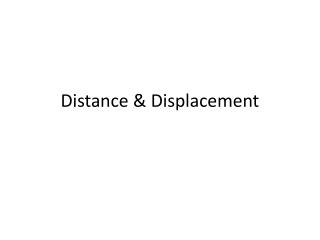# Distance & Displacement - PowerPoint PPT PresentationDownload PresentationDistance & Displacement

Distance & DisplacementDownload Presentation## Distance & Displacement

- - - - - - - - - - - - - - - - - - - - - - - - - - - E N D - - - - - - - - - - - - - - - - - - - - - - - - - - -
##### Presentation Transcript

1. Distance & Displacement

2. Distance is a Scalar • A measure of the length of a path an object takes in moving from one position to another is called distance. • Distance is how far an object has moved • Distance is a scalar quantity.

3. 0 1 2 3 4 5 6 7 8 9 10 cm Distance • Distance (d) = how far an object travels. • Does not depend on direction. • Imagine an ant crawling along a ruler. • What distance did the ant travel? d = 3 cm

4. 0 1 2 3 4 5 6 7 8 9 10 cm Distance • Distance does not depend on direction. • Now what distance did the ant travel? d = 3 cm

5. 0 1 2 3 4 5 6 7 8 9 10 cm Distance • Distance does not depend on direction. • Follow the ant again: • What distance did the ant walk this time? • d = 7 cm

6. Displacement is a Vector • Displacement is the change in position of an object and includes a description of the direction of motion. • Determined by drawing a straight line from starting position to ending position • Is not always equal to distance traveled.

7. Displacement

8. - + 0 1 2 3 4 5 6 7 8 9 10 cm Displacement • Displacement has direction. • Distance: 3 cm • Displacement: +3 cm The positive gives the ant a direction

9. - + 0 1 2 3 4 5 6 7 8 9 10 cm Displacement • Find the ant’s displacement again. • Distance: 3 cm • Displacement: -3 cm

10. - + 0 1 2 3 4 5 6 7 8 9 10 cm Displacement • Find the distance and displacement of the ant. • Distance: 7 cm • Displacement: +3 cm • = 5cm – 2cm = +3cm

11. QUESTION: How far (in km) will a car travel in 15 min at 20 m/s ? d = s x t = (20 m) x (60 s)  (15 min) s min = 18 000 m (divide 1000 for km) = 18 km

12. Displacement E.g. the distance travelled here by a driver was 14.5 km even though the displacement was only 2.7 km [North].

13. E.g If a person travels 40m east, turns and travels 30m west, the displacement of the person is 10m east. • If a person travels 40m east and then travels another 50m east, the displacement is 90m east.

14. Displacement Example: Mandisawalks 2 m [East] and then 1 m [West]. What was her distance travelled? What was her displacement? Distance travelled: Dd = 2 m + 1 m = 3 m Displacement: She is 1 m [East] of her original starting position. 1 m 1 m

15. Displacement 2 m We can represent [East] as the positive direction and [West] as the negative direction: = 2 m [East] + 1 m [West] = + 2 m – 1 m = + 1 m = 1 m [East] 1 m 1 m

16. What is the difference between distance and displacement? Distance: How far something travels Displacement: How far something travels in a given direction in a straight line from start to finish.

17. A soccer player changes position back and forth on the field. In the diagram below each position is marked where the player reverses direction. The player moves from position A to B to C to D. • Find the distance and displacement of travel.

18. Solution: Distance = 85 m Displacement = 55 m left of A

19. Displacement • Therefore, displacement =difference between an object’s final position and its starting position. • Does depend on direction. • Displacement = final position – initial position • Examples of directions: • + and – • N, S, E, W • Angles

20. Displacement vs. Distance • Example of distance: • The car drove 30 km. • Example of displacement: • The car drove 30 km. EAST. • An object’s distance traveled and its displacement aren’t always the same!

21. An athlete runs around a track that is 100 meters long three times, then stops. What is the athlete’s distance and displacement? Distance = 300 m Displacement = 0 m Why? Displacement vs. Distance

22. Complete worksheet